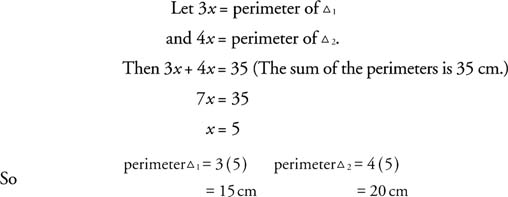## Similar Triangles: Perimeters and Areas

When two triangles are similar, the reduced ratio of any two corresponding sides is called the scale factor of the similar triangles. In Figure 1, Δ ABC∼ Δ DEF.Figure 1 Similar triangles whose scale factor is 2 : 1.

The ratios of corresponding sides are 6/3, 8/4, 10/5. These all reduce to 2/1. It is then said that the scale factor of these two similar triangles is 2 : 1.

The perimeter of Δ ABC is 24 inches, and the perimeter of Δ DEF is 12 inches. When you compare the ratios of the perimeters of these similar triangles, you also get 2 : 1. This leads to the following theorem.

Theorem 60: If two similar triangles have a scale factor of a : b, then the ratio of their perimeters is a : b.

Example 1: In Figure 2, Δ ABC∼ Δ DEF. Find the perimeter of Δ DEFFigure 2 Perimeter of similar triangles.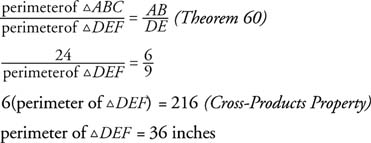Figure 3 shows two similar right triangles whose scale factor is 2 : 3. Because GH ⊥ GI and JK ⊥ JL , they can be considered base and height for each triangle. You can now find the area of each triangle.Figure 3 Finding the areas of similar right triangles whose scale factor is 2 : 3.Now you can compare the ratio of the areas of these similar triangles.This leads to the following theorem:

Theorem 61: If two similar triangles have a scale factor of a : b, then the ratio of their areas is a2 : b2.

Example 2: In Figure 4, Δ PQR∼ Δ STU. Find the area of Δ STU.Figure 4 Using the scale factor to determine the relationship between the areas of similar triangles.

The scale factor of these similar triangles is 5 : 8.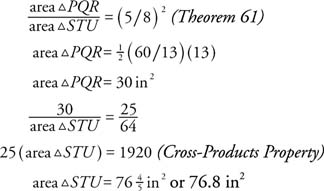Example 3: The perimeters of two similar triangles is in the ratio 3 : 4. The sum of their areas is 75 cm2. Find the area of each triangle.

If you call the triangles Δ1 and Δ2, then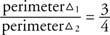According to Theorem 60, this also means that the scale factor of these two similar triangles is 3 : 4.Because the sum of the areas is 75 cm2, you get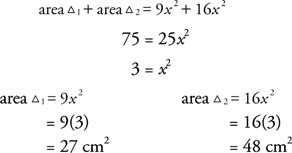Example 4: The areas of two similar triangles are 45 cm2 and 80 cm2. The sum of their perimeters is 35 cm. Find the perimeter of each triangle.

Call the two triangles Δ1 and Δ2 and let the scale factor of the two similar triangles be a : b.a : b is the reduced form of the scale factor. 3 : 4 is then the reduced form of the comparison of the perimeters.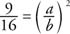Reduce the fraction.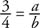Take square roots of both sides.